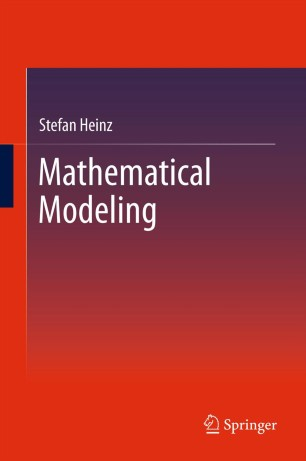# Mathematical ModelingTextbook

1. Front Matter
Pages I-XVI
2. Stefan Heinz
Pages 1-38
3. Stefan Heinz
Pages 39-74
4. Stefan Heinz
Pages 75-114
5. Stefan Heinz
Pages 115-164
6. Stefan Heinz
Pages 165-205
7. Stefan Heinz
Pages 207-246
8. Stefan Heinz
Pages 247-293
9. Stefan Heinz
Pages 295-334
10. Stefan Heinz
Pages 335-390
11. Stefan Heinz
Pages 391-440
12. Back Matter
Pages 441-460

### Introduction

The whole picture of Mathematical Modeling is systematically and thoroughly explained in this text for undergraduate and graduate students of mathematics, engineering, economics, finance, biology, chemistry, and physics. This textbook gives an overview of the spectrum of modeling techniques, deterministic and stochastic methods, and first-principle and empirical solutions.

Complete range: The text continuously covers the complete range of basic modeling techniques: it provides a consistent transition from simple algebraic analysis methods to simulation methods used for research. Such an overview of the spectrum of modeling techniques is very helpful for the understanding of how a research problem considered can be appropriately addressed.

Complete methods: Real-world processes always involve uncertainty, and the consideration of randomness is often relevant. Many students know deterministic methods, but they do hardly have access to stochastic methods, which are described in advanced textbooks on probability theory. The book develops consistently both deterministic and stochastic methods. In particular, it shows how deterministic methods are generalized by stochastic methods.

Complete solutions: A variety of empirical approximations is often available for the modeling of processes. The question of which assumption is valid under certain conditions is clearly relevant. The book provides a bridge between empirical modeling and first-principle methods: it explains how the principles of modeling can be used to explain the validity of empirical assumptions. The basic features of micro-scale and macro-scale modeling are discussed – which is an important problem of current research.

#### Authors and affiliations

1. 1.Dept. MathematicsUniversity of WyomingLaramieUSA

Dr. Stefan Heinz is a Professor of Mathematics at the University of Wyoming. He holds a Ph.D. in Physics from the Heinrich-Hertz Institute, Berlin. His research interests are in mathematical modeling, multiscale processes, stochastic analysis, Monte Carlo simulations, computational fluid dynamics, turbulence, combustion, and multiphase flows. He has authored more than seventy refereed publications and the textbook Statistical Mechanics of Turbulent Flows (Springer, 2003). For more than ten years he has taught a variety of courses: calculus, probability, ordinary, partial, and stochastic differential equations, applied mathematics, and deterministic and stochastic mathematical modeling. His exceptional teaching was awarded in 2007 by the College of Arts and Sciences Extraordinary Merit in Teaching Award. In 2008 he was honored as Adjunct Professor of Mechanical Engineering. He has held visiting professor appointments at ETH Zurich, Delft Technical University, and the National Center for Atmospheric Research (NCAR) at Boulder.

### Bibliographic information

• Book Title Mathematical Modeling
• Authors Stefan Heinz
• DOI https://doi.org/10.1007/978-3-642-20311-4
• Copyright Information Springer-Verlag Berlin Heidelberg 2011
• Publisher Name Springer, Berlin, Heidelberg
• eBook Packages Engineering Engineering (R0)
• Hardcover ISBN 978-3-642-20310-7
• Softcover ISBN 978-3-642-44388-6
• eBook ISBN 978-3-642-20311-4
• Edition Number 1
• Number of Pages XVI, 460
• Number of Illustrations 0 b/w illustrations, 0 illustrations in colour
• Topics
• Buy this book on publisher's site

## Reviews

From the reviews:

“It is of special interest for a fluid dynamics audience since most types of models one would like to apply in fluid dynamic systems are introduced. … Mathematical modeling is of great use as background reading material for any course on advanced fluid dynamics. … A total of 570 exercise questions are organized in 220 problems … . I strongly recommend the book to anyone wanting to acquire good understanding what mathematical modeling is about.” (Dirk J. E. M. Roekaerts, Theoretical and Computational Fluid Dynamics, Vol. 27, 2013)

“The book covers two primary types of modeling: deterministic and stochastic. … The text can be used for a course at the undergraduate or graduate level. … This course would be particularly beneficial to students outside of science and engineering. … I like this book and recommend it as a text in mathematical modeling. I also recommend it for those studying turbulent fluid mechanics, stochastic vibration & structures, noise, meteorology, random data management, and stock market analysis.” (Peyman Givi, AIAA Journal, Vol. 50 (12), December, 2012)

“This book is aimed at advanced undergraduate and graduate students in mathematics who have a background in single and multivariable calculus, linear algebra, and ordinary differential equations. … focuses on the construction of mathematical models, the derivation of analytical solutions to tractable models, and the qualitative analysis of the behavior of solutions to the less tractable models. … The entire book could be covered in a two-semester sequence of courses at the graduate level. Summing Up: Recommended. Upper-division undergraduates and graduate students.” (B. Borchers, Choice, Vol. 49 (5), January, 2012)

“The book under review is aimed at students in mathematics, physics, engineering, biology, chemistry, economics, finance. … well-written, generously illustrated, and contains many carefully explained examples that are often based on real data. Each chapter starts with a brief ‘Motivation’ section and concludes with a ‘Summary’ that emphasizes the most important ideas and techniques. … This textbook nicely complements existing literature on mathematical modeling and can be used both as a main source or as a supplementary text … in applied mathematics and mathematical modeling.” (Svitlana P. Rogovchenko, Zentralblatt MATH, Vol. 1239, 2012)

“It is a collection of examples of models … with a large emphasis on engineering, the author’s major field as well as the theme of its Springer series. … this book may serve as a reference for those seeking examples of modeling in different specializations, or as a resource for exercises; several of the latter are at the end of each chapter.” (J. David Logan, SIAM Review, Vol. 54 (3), 2012)